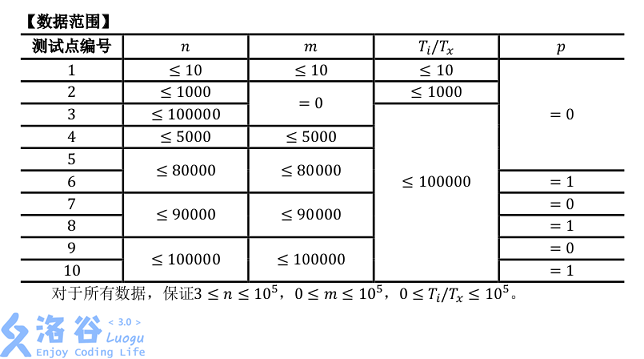# P4425 [HNOI/AHOI2018]转盘

• 258通过
• 537提交
• 题目提供者 M_sea
• 评测方式 云端评测
• 标签 枚举,暴力 线段树 各省省选 2018 安徽 湖南 高性能
• 难度 NOI/NOI+/CTSC
• 时空限制 2000ms / 512MB
• 提示：收藏到任务计划后，可在首页查看。

## 题目描述

一次小G和小H准备去聚餐，但是由于太麻烦了于是题面简化如下：

一个转盘上有摆成一圈的$n$个物品（编号1~$n$），其中的$i$个物品会在$T_i$时刻出现。

在0时刻时，小G可以任选$n$个物品中的一个，我们将其编号为$s_0$。并且如果$i$时刻选择了物品$s_i$，那么$i+1$时刻可以继续选择当前物品或选择下一个物品。当$s_i$为$n$时，下一个物品为物品$1$，否则为物品$s_{i}+1$。在每一时刻（包括0时刻），如果小G选择的物品已经出现了，那么小G将会标记它。小H想知道，在物品选择的最优策略下，小G什么时候能标记所有物品？

但麻烦的是，物品的出现时间会不时修改。我们将其描述为$m$次修改，每次修改将改变其中一个物品的出现时间。每次修改后，你也需求出当前局面的答案。对于其中部分测试点，小H还追加了强制在线的要求。

## 输入输出格式

输入格式：

第一行三个非负整数$n$、$m$、$p$，代表一共有$n$个物品，$m$次修改。$p$只有0或1两种取值，强制在线时$p$为1，否则$p$为0.

接下来一行，有$n$个非负整数，第$i$个数$T_i$代表物品$i$的出现时间。

接下来$m$行，每行两个非负整数$x$、$y$，代表一次修改及询问。修改方式如下：

（1）如果$p=0$，则表示物品$x$的出现时间$T_x$修改为$y$。

（2）如果$p=1$，在先将$x$和$y$分别异或$LastAns$，得到$x'$和$y'$，然后将物品$x'$的出现时间$T_{x'}$修改为$y'$。其中的$LastAns$是前一个询问的结果；特别的，第一次修改时$LastAns$为初始局面的答案。

保证输入合法。

输出格式：

第一行一个整数，代表初始局面的答案。

接下来$m$行每行一个整数，分别代表每次修改后的答案。

## 输入输出样例

输入样例#1： 复制
5 3 0
1 2 3 4 5
3 5
5 0
1 4
输出样例#1： 复制
5
7
6
7

## 说明

【数据范围】$3≤n≤10^5,0≤m≤10^5,0≤T_i/T_x≤10^5$。

提示
标程仅供做题后或实在无思路时参考。
请自觉、自律地使用该功能并请对自己的学习负责。
如果发现恶意抄袭标程，将按照I类违反进行处理。